This is a Clilstore unit. You can .

# Solid figures

Solid figures are three-dimensional. Prism: A prism is a figure made of two parallel faces that are polygons of the same shape and sides that are parallelograms.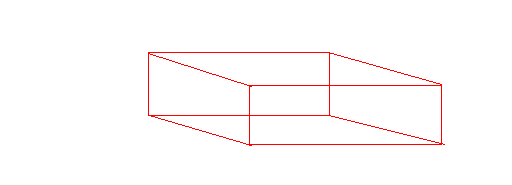Triangular prism: A prism with triangular faces. The following is a triangular prism.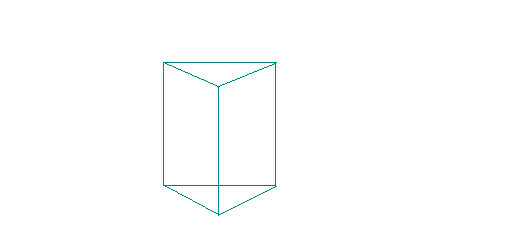Cube: A cube is a prism with squares for sides and faces. The following is a cube.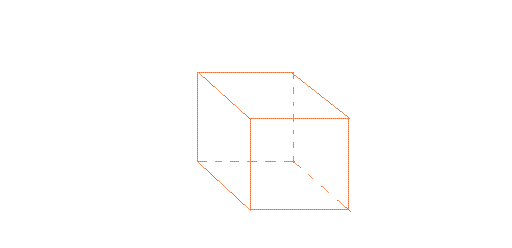Pyramid: A figure with a base that is a polygon and triangular sides. The following is a pyramid.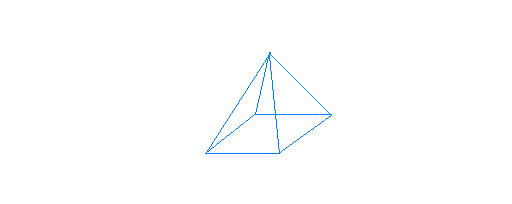Cone: A cone has a circular base connected to a vertex.The following is a cone.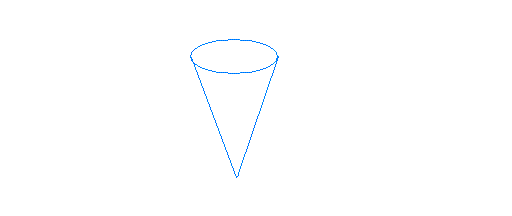Cylinder: A cylinder has two equal circular bases that are parallel.The following is a cylinder.Sphere: A figure with a curved surface in which all points on the surface are equal distance from the center.The following is a sphere.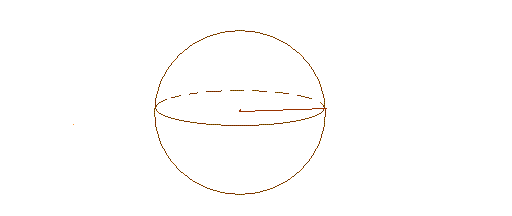Short url:   https://clilstore.eu/cs/3608